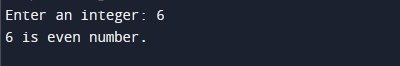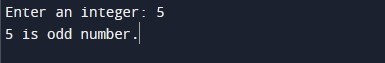# C Program to find number is even or odd

In this tutorial you will learn about the C Program to find number is even or odd and its application with practical example.

In this tutorial, we will learn to create a program to find whether a given number is even or odd using C programming.

Prerequisites

Before starting with this tutorial we assume that you are best aware of the following C programming topics:

• C Operators
• C if else statement

## How to find number is even or odd

An integer number that is exactly divisible by 2 is known as even number, example: 0, 8, -24. Programmatically, we can check number is even or add using modulus (%) operator. If modulus 2 of any integer number is equals to 0 then, the number is even otherwise odd.

## Program to find number is even or odd

In this program we will find a number entered by user is even or odd using modulus (%) operator. We would first declared and initialized the required variables. Next, we would prompt user to input an integer number. Later in the program we will check number entered by user is even or odd using modulus (%) operator. We will then display message number is even or odd.

Output 1:-Output 2:-In the above program, we have first declared and initialized a set variables required in the program.

• num = it holds the input integer number

In the next statement user will be prompted to enter an integer number to check is assigned to variable ‘num’. In the next statement we check modulus 2 of integer number is equals to 0 or not i.e. `if(num % 2 == 0)` then the number is even otherwise odd. Now, we will be displaying the number is even or odd.

## Program to check number is even or odd Using Bitwise operator

In this program we will check a number entered by user is even or odd using bitwise(&) operator. We would first declared and initialized the required variables. Next, we would prompt user to input an integer number. Later in the program we will check number entered by user is even or odd using bitwise(&) operator. We will then display message number is even or odd.

Output 1:-Output 2:-In the above program, we have first declared and initialized a set variables required in the program.

• num = it holds the input integer number

In the next statement user will be prompted to enter an integer number to check is assigned to variable ‘num’. In the next statement we check number is even or odd using bitwis(&) operator i.e. `if(num & 1 == 1)` then the number is odd otherwise even. Now, we will be displaying the number is even or odd.

In this tutorial we have learn about the C Program to find number is even or odd and its application with practical example. I hope you will like this tutorial.